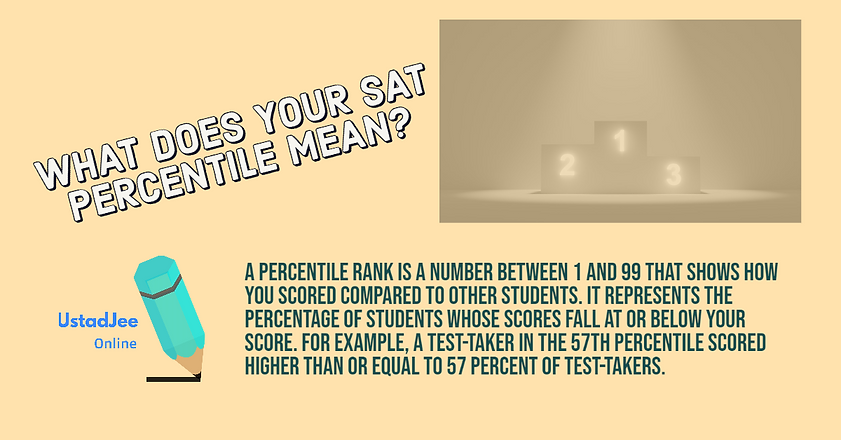## SAT#### Algebra 1

• Linear expression in one variable

• Linear inequalities in one variable

• The linear relationship between two quantities

#### Algebra 2

• Linear inequalities in two variables

• Linear equations in two variables

• Algebraically solve linear equations in one variable

• Algebraically solve systems of two linear equations in two variables

• Understand the connection between algebraic and graphical representations

# Heart of Algebra

#### PSDA 1

• Ratio and Proportions

• Rates

• Practice problems and Solution

#### PSDA 2

• Solve single- and multi-step problems involving percentages , measurement quantities, units, and unit conversion.

• Given a scatter plot, use linear, quadratic, or exponential models to describe how the variables are related

• Compare linear growth with exponential growth.

• Use two-way tables to summarize categorical data and relative frequencies, and calculate conditional probability

• Make inferences about population parameters based on sample data

• Use statistics to investigate measures of the center of data and analyze shape, center, and spread.

• Evaluate reports to make inferences, justify conclusions, and determine the appropriateness of data collection methods

# Problem Solving and Data Analysis (PSDA)

#### PAM 1

• Create a quadratic or exponential function

• Determine the most suitable form of expression

• Create equivalent expressions involving rational exponents

• Create an equivalent form of an algebric expression

#### PAM 2

• Add, subtract, and multiply polynomial expressions and simplify the result. The expressions will have rational coefficients

• Solve an equation in one variable that contains radicals or contains the variable in the denominator of a fraction

#### PAM 3

• Solve a system of one linear equation and one quadratic equation

• Rewrite simple rational expression

• Interpret parts of nonlinear expressions in terms of their context

• Understand the relationship between zeros and factors of polynomials

#### PAM 4

• Understand a nonlinear relationship between two variables

• Use function notation, and interpret statements using function notation. The student will use function notation to solve conceptual problems related to transformations and compositions of functions

• Use structure to isolate or identify a quantity of interest in an expression or isolate a quantity of interest in an equation

#### PAM 5

• Use structure to isolate or identify a quantity of interest

• In an expression, isolate a quantity of interest in an equation

• The student will rearrange an equation or formula to isolate

# Passport to Advance Mathematics (PAM)

## Additional Topics in SAT Maths

We are here to make a difference

#### Topics Covered

• Solve problems using volume formulas,length of a side, area of a face, or volume of a solid, to calculate missing information

• Use trigonometric ratios and the Pythagorean theorem to solve applied problems involving right triangles

• Convert between degrees and radians and use radians to determine arc lengths

• Apply theorems about circles to find arc lengths, areas of sectors, angle measures and chord lengths

• Use theorems about congruence and similarity to solve problems about lines, angles, and triangles. Use the relationship between similarity, right triangles, and trigonometric ratios.

• Create or use an equation in two variables to solve a problem about a circle in the coordinate plane.

• Use the relationship between sine and cosine of complementary angles